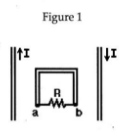# Problem: In Figure 1, two parallel wires carry a current I in opposite directions. A rectangular loop is midway between the wires. The current I is decreasing. The induced current through the resistor R is:  A) from b to a B) from a to b C) zero

###### FREE Expert Solution
91% (257 ratings)
###### Problem Details

In Figure 1, two parallel wires carry a current I in opposite directions. A rectangular loop is midway between the wires. The current I is decreasing. The induced current through the resistor R is:

A) from b to a

B) from a to b

C) zero